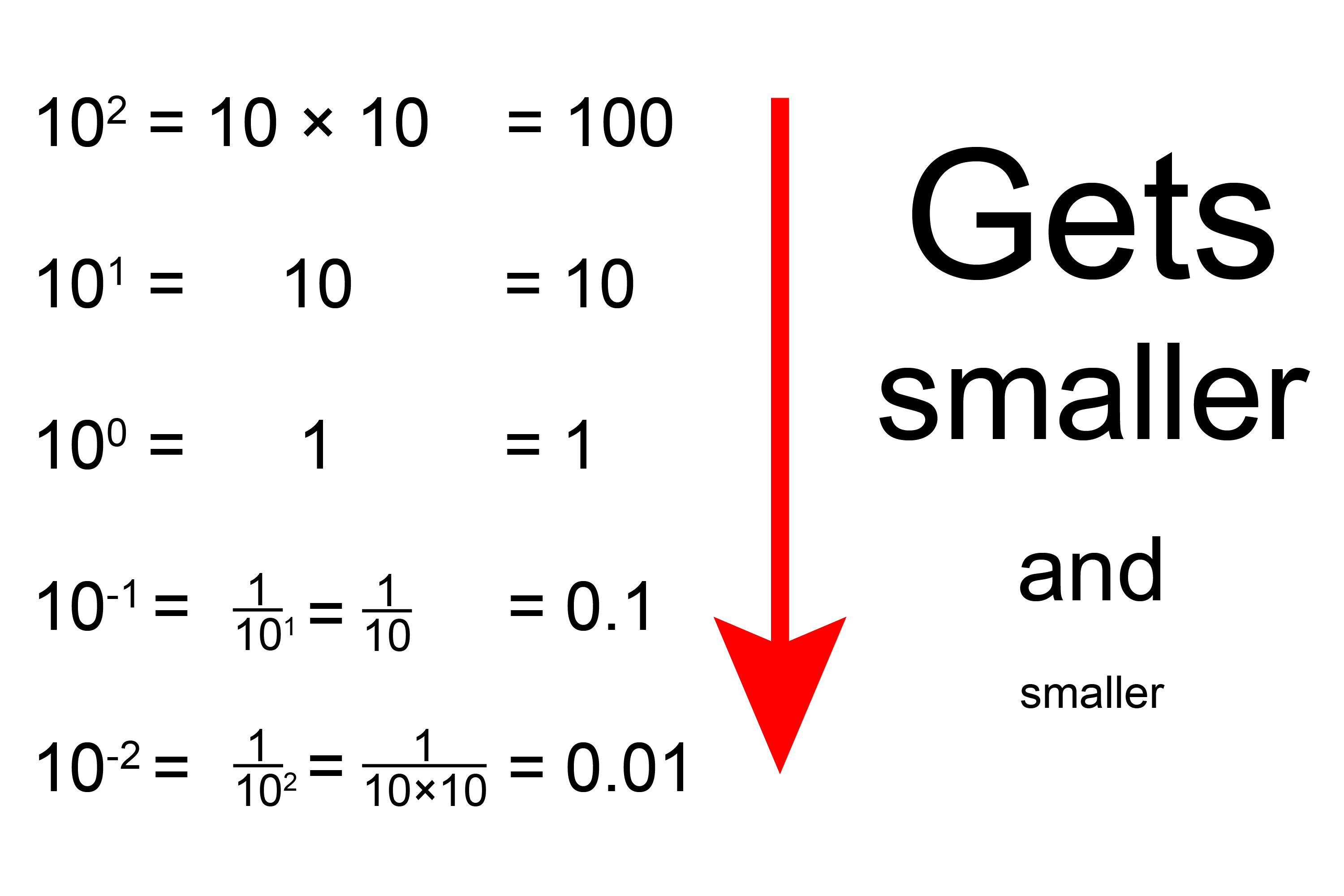# Indices law - law 2

## a^-n=1/a^nTo remember this put simple numbers in. ie Try simple numbers you know first.

10^-2=1/10^2=1/(10times10)=1/100=0.01

8^-1=1/8=0.125

8^-2=1/8^2=1/(8times8)=1/64=0.0156

Also try other examples you know like starting with 10^2 and remove 1 from the power and understand the answer you get:Examples

1.       5^-3  is the same as  1/5^3

2.       x^-3  is the same as  1/x^3